Euler's Method for ordinary differential equations using Mathematica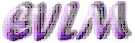Аnotation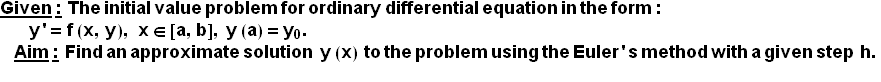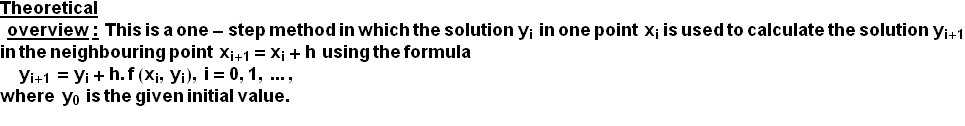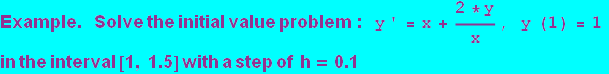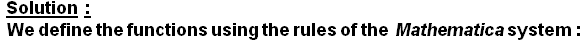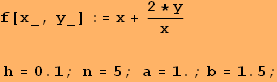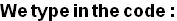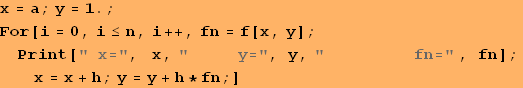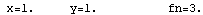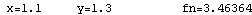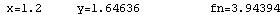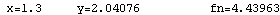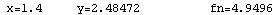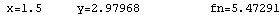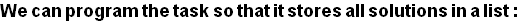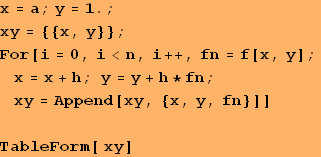1 1 1.1 1.3 3. 1.2 1.64636 3.46364 1.3 2.04076 3.94394 1.4 2.48472 4.43963 1.5 2.97968 4.9496

Conclusion:   The solution derived by using the Euler's method is in the form of a table of the variable function y(x), displayed in the second column of the table above. Since the apriori error of the method is О(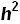) and here h=0.1, then these solutions must be taken rounded to only two symbols past the decimal point.

Graphic of the solution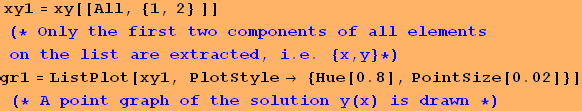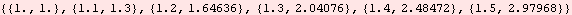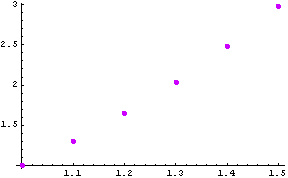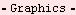Created by Wolfram Mathematica 6.0  (22 September 2008)